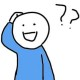Ascii art是一种使用连续排列的ascii字符进行图形设计的技术。它可以显示在任意的文本框中。ascii art出现于上世纪70年代，最初是当时电脑显示技术不发达时用于显示简单图像的一种娱乐。后来逐渐流行开来，有了专门以此为兴趣的艺术家和研究者。

⣿⣿⣿⣿⣿⣿⢟⣡⣴⣶⣶⣦⣌⡛⠟⣋⣩⣬⣭⣭⡛⢿⣿⣿⣿⣿
⣿⣿⣿⣿⠋⢰⣿⣿⠿⣛⣛⣙⣛⠻⢆⢻⣿⠿⠿⠿⣿⡄⠻⣿⣿⣿
⣿⣿⣿⠃⢠⣿⣿⣶⣿⣿⡿⠿⢟⣛⣒⠐⠲⣶⡶⠿⠶⠶⠦⠄⠙⢿
⣿⠋⣠⠄⣿⣿⣿⠟⡛⢅⣠⡵⡐⠲⣶⣶⣥⡠⣤⣵⠆⠄⠰⣦⣤⡀
⠇⣰⣿⣼⣿⣿⣧⣤⡸⢿⣿⡀⠂⠁⣸⣿⣿⣿⣿⣇⠄⠈⢀⣿⣿⠿
⣰⣿⣿⣿⣿⣿⣿⣿⣷⣤⣈⣙⠶⢾⠭⢉⣁⣴⢯⣭⣵⣶⠾⠓⢀⣴
⣿⣿⣿⣿⣿⣿⣿⣿⣿⣿⣿⣿⣉⣤⣴⣾⣿⣿⣦⣄⣤⣤⣄⠄⢿⣿
⣿⣿⣿⣿⣿⣿⣿⣿⠿⠿⠿⠿⣿⣿⣿⣿⣿⣿⣿⣿⣿⣿⣿⣇⠈⢿
⣿⣿⣿⣿⣿⣿⡟⣰⣞⣛⡒⢒⠤⠦⢬⣉⣉⣉⣉⣉⣉⣉⡥⠴⠂⢸
⠻⣿⣿⣿⣿⣏⠻⢌⣉⣉⣩⣉⡛⣛⠒⠶⠶⠶⠶⠶⠶⠶⠶⠂⣸⣿
⣥⣈⠙⡻⠿⠿⣷⣿⣿⣿⣿⣿⣿⣿⣿⣿⣾⣿⠿⠛⢉⣠⣶⣶⣿⣿
⣿⣿⣿⣶⣬⣅⣒⣒⡂⠈⠭⠭⠭⠭⠭⢉⣁⣄⡀⢾⣿⣿⣿⣿⣿⣿

## 在R#语言之中使用ASCII Art函数require(graphics2D);

"1537192287563.jpg"
|> resizeImage(factor = 1)
|> asciiArt
|> writeLines(con = "bilibili.txt")
;## ASCII Art的方法实现

''' <summary>
''' convert bitmap to ascii characters
''' </summary>
''' <param name="image"></param>
''' <returns></returns>
<ExportAPI("asciiArt")>
<RApiReturn(GetType(String))>
Public Function asciiArt(image As Object, Optional charSet As String = "+-*.", Optional env As Environment = Nothing) As Object
Dim bitmap As Image

If image Is Nothing Then
Return Internal.debug.stop("the required bitmap data can not be nothing!", env)
ElseIf TypeOf image Is Image Then
bitmap = DirectCast(image, Image)
ElseIf TypeOf image Is Bitmap Then
bitmap = CType(DirectCast(image, Bitmap), Image)
Else
Return Message.InCompatibleType(GetType(Bitmap), image.GetType, env)
End If

Dim font As New Font(FontFace.Consolas, 10)
Dim pixels As WeightedChar() = charSet.GenerateFontWeights(font)

Return bitmap _
.GetBinaryBitmap() _
.Convert2ASCII(pixels)
End Function

## 图像至ASCII字符串转换的原理

''' <summary>
''' a pixel char
''' </summary>
Public Class WeightedChar

''' <summary>
''' a char that represent a pixel on the source bitmap
''' </summary>
''' <returns></returns>
Public Property Character As String
Public Property CharacterImage As Bitmap
''' <summary>
''' the gray scale value
''' </summary>
''' <returns></returns>
Public Property Weight As Double

Public Overrides Function ToString() As String
Return \$"{Character} ({Weight})"
End Function

<MethodImpl(MethodImplOptions.AggressiveInlining)>
Friend Shared Function getDefaultCharSet() As [Default](Of  WeightedChar())
Return CharSet.GenerateFontWeights()
End Function
End Class

### 建立映射关系

<Extension>
Private Function GetWeight(c As Char, size As SizeF) As Double
Dim charImage = HelperMethods.DrawText(c.ToString(), Color.Black, Color.White, size)
Dim totalsum As Double = 0

Using btm As BitmapBuffer = BitmapBuffer.FromImage(charImage)
For i As Integer = 0 To btm.Width - 1
For j As Integer = 0 To btm.Height - 1
Dim pixel As Color = btm.GetPixel(i, j)
totalsum += (CInt(pixel.R) + CInt(pixel.G) + CInt(pixel.B)) \ 3
Next
Next
End Using

' Weight = (sum of (R+G+B)/3 for all pixels in image) / Area. (Where Area = Width*Height )
End Function

### 转换图像为字符串

* ALGORITHM:
*
*  1- Get target Image size (w=Width,h=Height)
*  2- Create Result Image with white background and size W = w*character_image_width
*                                                        H = h*character_image_height
*  3- Create empty string to hold the text
*
*  4- for (int j=0;j=target_Image_Height;j++)  --> ALL ROWS
*       5- Create row text string
*       for (int i=0;i=target_Image_Width;i++) --> ALL COLUMNS
*          6- Get target pixel weight
*          7- Get closest weight from character list
*          8- Paste character image in position w = i*character_image_width
*                                               h = j*character_image_height
*            ¡¡ Be careful with coordinate system when placing Images !!
*          9- Add (string)character to row text string
*       10- Add row text string to text holding string
*  11 - return resulting Image & Text

Using blackAndWhite As BitmapBuffer = BitmapBuffer.FromImage(monoImage)
For j As Integer = 0 To monoImage.Height - 1
Dim line As New List(Of String)() From {}

For i As Integer = 0 To monoImage.Width - 1
' COLUMN
Dim pixel As Color = blackAndWhite.GetPixel(i, j)
Dim targetvalue As Double = (CInt(pixel.R) + CInt(pixel.G) + CInt(pixel.B)) \ 3
Dim closestchar As WeightedChar = characters _
.Where(Function(t)
Return stdNum.Abs(t.Weight - targetvalue) = characters.Min(Function(e) stdNum.Abs(e.Weight - targetvalue))
End Function) _
.FirstOrDefault()

Next

Call out.WriteLine(line.JoinBy(""))
Next
End Using
Latest posts by 谢桂纲 (see all)

### Order by Date Name Attachments

1.2.3.4.5.